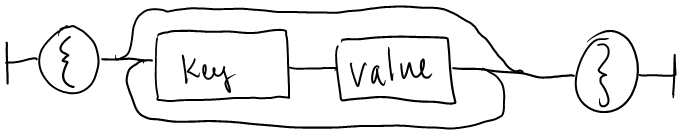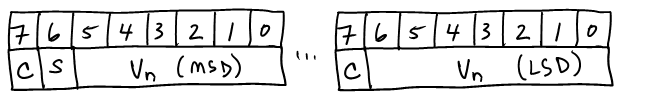﻿ Introducing PBON

# Introducing PBON

PBON (Portable Binary Object Notation) is a light-weight binary data interchange format inspired by JSON.

PBON is built on the same structures as JSON, namely:

• A collection of key/value pairs (realized as an object in some programming languages)
• An ordered list of values (realized as an array in some programming languages)

The primary difference between JSON and PBON is that PBON is a more compact format that allows binary data (including Unicode strings) to be represented directly in the encoding without escaping. The trade-off is that PBON loses some human-readability to achieve this compactness.

PBON prefers the simplicity of JSON over implementing the most compact binary representation possible, in order to maintain some human-readability and to make implementation straightforward.

PBON supports backward compatibility by enabling implementations to skip values with unrecognized keys, for example, key/value pairs added as part of a newer version of an object.

In PBON, an object is an unordered set of key/value pairs. An object begins with a left brace { and ends with a right brace }.

objectA key is a positive integer encoded as a variable-length integer.

An array is an ordered collection of values. An array begins with a left bracket [ and ends with a right bracket ].

arrayA value can be binary, a string, an integer, a float, an object, an array, true (t) or false (f) or null (~).

valueA binary value is a length-prefixed sequence of bytes.

A length-prefix is a non-negative integer value, encoded as variable-length integer.

A string is a length-prefixed sequence of UTF-8 encoded characters.

An integer is a length-prefixed base-256 encoded value in big-endian order. Negative integer values are stored using the bitwise complement of the negative value with the most significant bit (the sign bit) set to 1.

A float is a length-prefixed IEEE 754 encoded floating point value in big-endian order.

## Variable-length integer

A variable-length integer is stored as an initial byte followed by one or more trailing bytes.

The most-significant bit of each byte (C) is a continuation indicator, which is set to 1 for all but the last byte.

The second most-significant bit of the first byte (S) is reserved as the sign indicator, which is set to 1 for negative values.

The remaining bits (Vn) contain the value in big-endian byte order (most significant digits first).Negative values are transformed using a bitwise complement operation before encoding. This ensures that small negative numbers will also occupy a small amount of encoded space.

For example, here's the value 1. It's a single byte so the continuation bit (C) is not set, and it's a positive value so the sign bit (S) is not set:

```0000 0001
```

And here's the value 300:

```1000 0010 0010 1100
```

To calculate this value, start with the binary encoding of 300 and split it into 7-bit groups starting from the least-significant digit:

```300 → 10 0101100
```

And finally, set the sign bit (S) to 0 to indicate a positive value and the continuation bit of each byte (C) except the last to 1:

```→ 10000010 00101100
```

Here's the value -300:

```1100 0010 0010 1011
```

To encode a negative number, start with the binary encoding of the value, take the bitwise complement, and split it into 7-bit groups starting from the least-significant digit:

```   -300 → 1111 1110 1101 0100
~(-300) →         1 0010 1011
→          10 0101011
```

And finally, set the sign bit (S) to 1 to indicate a negative value and the continuation bit of each byte (C) except the last to 1:

```→ 11000010 00101011
```

## A complete example

```class Message1
{
string Name = "Foo";
}
```

This message would be encoded as the following bytes:

```7B 01 03 46 6F 6F 7D
```

Let's break this down:

The first and last bytes (7B ... 7D) are the braces { } that surround every object.

The second byte (01) is the first member key (1) encoded as a variable-length integer.

The third byte (03) is the length of the string member value to follow (3 bytes), also encoded as a variable-length integer.

And finally, bytes 4-6 are the UTF-8 encoded bytes of the string (46 6F 6F).

Now let's add a second member with an integer value:

```class Message2
{
string Name = "Foo";
int Score = 100;
}
```

This message could be encoded as the following bytes:

```7B 01 03 46 6F 6F 02 01 64 7D
```

The 7th byte (02) is the new member key (2) encoded as a variable-length integer.

The 8th byte (01) is the length of the integer member value to follow (1 byte), also encoded as a variable-length integer.

And finally, the 9th byte (64) is the base-256 encoded value 100.

Now let's say that we want an array of scores:

```class Message3
{
string Name = "Foo";
int[] Scores = new int[] { 1, 2, 3 };
}
```

This message could be encoded as the following bytes:

```7B 01 03 46 6F 6F 03 5B 01 01 01 02 01 03 5D 7D
```

The 7th byte (03) is the new member key (3) encoded as a variable-length integer.

Note that we chose 3 as the member key so as not to conflict with the definition of the previous Message2 class and possibly break backward compatibility.

The 9th and 16th bytes (5B ... 5D) are the brackets [ ] that surround every array.

Bytes 10-15 are the values in the array, each of which is the length of the encoded value (encoded as a variable-length integer) followed by the integer value (encoded as base 256).

## ABNF

```object = "{" members "}" / "{}" / null
members = pair / pair members
pair = key value
key = varint ; > 0
array = "[" elements "]" / "[]" / null
elements = value / value elements
value = binary / string / integer / float / object / array / true / false / null
-----
binary = length octets
length = varint ; >= 0
string = length utf-8 ; length is utf-8 octet count
integer = length base-256 ; big endian
float = length ieee-754 ; big endian
true = "t"
false = "f"
null = "~"
varint = variable-length-integer ; Big-endian variable-length quantity, continuation bit in MSB of each octet, sign in bit 6 of first octet
```